# PWM centered vs. left-aligned mode

Puls-Width-Modulation (PWM) can be used to implement a simple digital-to-analog converter to create analog signals with a microcontroller. There exist two modes of PWM, a left aligned asymmetric mode and a centered symmetric mode.

In left aligned mode (also called Fast PWM), the timer/counter counts from 0 to its maximum (e.g. 255 for a 8bit timer) and resets to zero when the maximum is reached (a so called overflow). If we imagine plotting the counter value w.r.t. time, it would look like a sawtooth signal.

In centered mode (also called Phase correct PWM), the timer/counter counts again from 0 to its maximum, and then instead of resetting, counts downward until it reaches zero. Thus, the counter value over time would look like a triangle signal.

Now to create the PWM signal in both modes, the counter value is compared with a compare-value which corresponds to the duty-cycle. When this value is either smaller or larger than the current timer value, the output is set HIGH or LOW. So basically the duty-cycle is the ratio between the time that the output is HIGH and the total period. In the following diagram we can see an example both modes with duty-cycles of 1/4, 1/2, 3/4 and 1.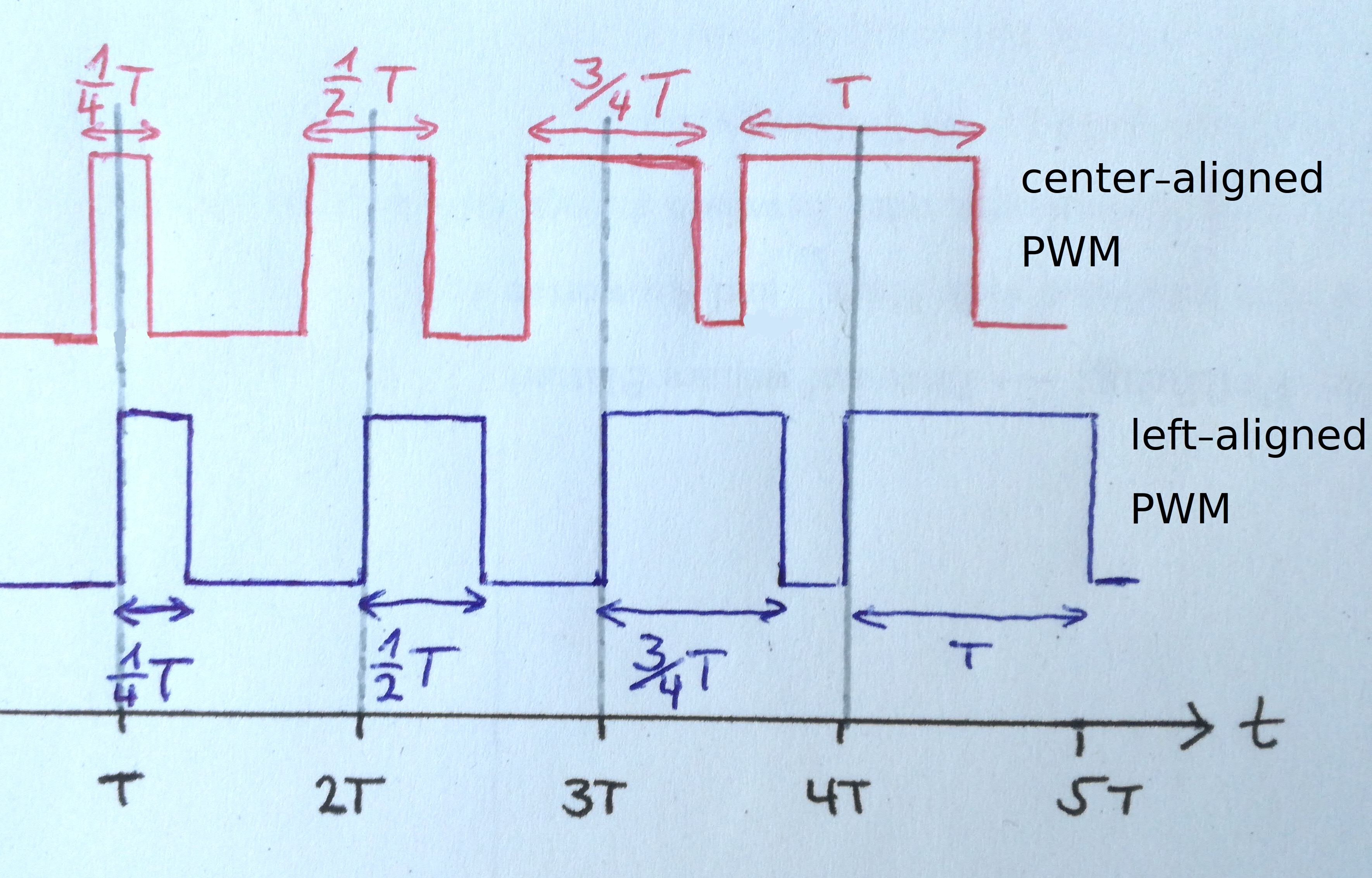Now to create an analog signal from this digital PWM signal, we use a low pass filter. This filter dampens high frequency content in the signal and lets low frequency content pass through. Our digital signal has instantaneous changes, which have (theoretically) and infinitely large frequency. So those parts will be dampened, and low frequency parts, like the DC average (which is a frequency of 0) pass through the filter. So let’s take a look at an example: We want to use this method to create an audio signal which lies in the range between 0 and 16kHz (this is approximately the human audible range). This means that we have to filter out all frequencies >16kHz and let the frequencies <16kHz pass trough undampened. But in reality such a filter doesn’t exist. For example if we build a simple RC-filter with a resistor and a capacitor, this is a first order filter. This means that the dampening of the filter augments with 20dB per decade (20dB = a ratio of 10). E.g. if we choose the values for R and C so that the corner frequency is around 16kHz, frequencies of 16kHz will be dampened by a factor of 1.41. Frequencies ten times higher, i.e. with 160kHz will be dampened by a factor of 10, 1600kHz by a factor of 100 and so on. We can build better filters, e.g. of second order with 40dB=100 per decade, but in any case we can get bette results if the frequency of our PWM signal is way larger than the maximum frequency of the analog signal we want to create.

Now in this post I will explore the difference between using the centered PWM mode versus the left-aligned mode to create an analog signal. For this purpose I created a model of two PWM-generators and an analog filter with MATLAB/Simulink. You can find the model and the script here if you want to play around with it and have access to MATLAB/Simulink. The model looks like this: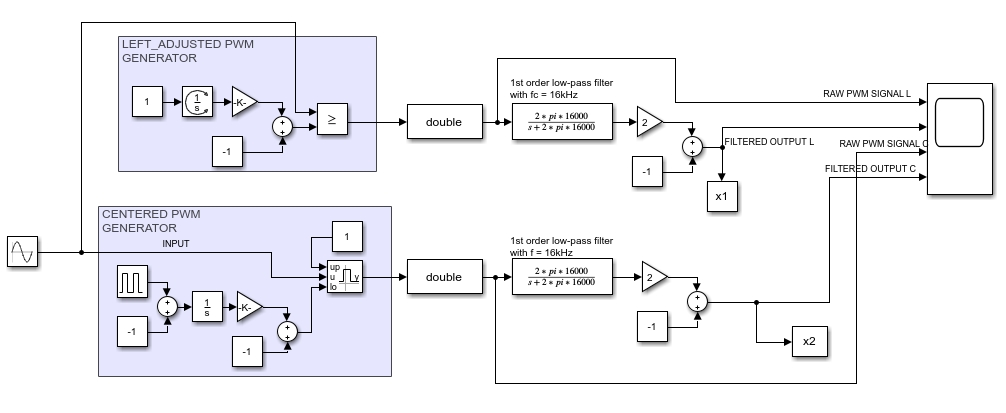The input signal is a sine wave with a frequency of 5kHz, the PWM frequency is 70kHz. The filter is of 1st order with a corner frequency of 16kHz. Here we can see the PWM signal and the filtered analog signal for both modes (L = left-aligned mode, C = centered mode).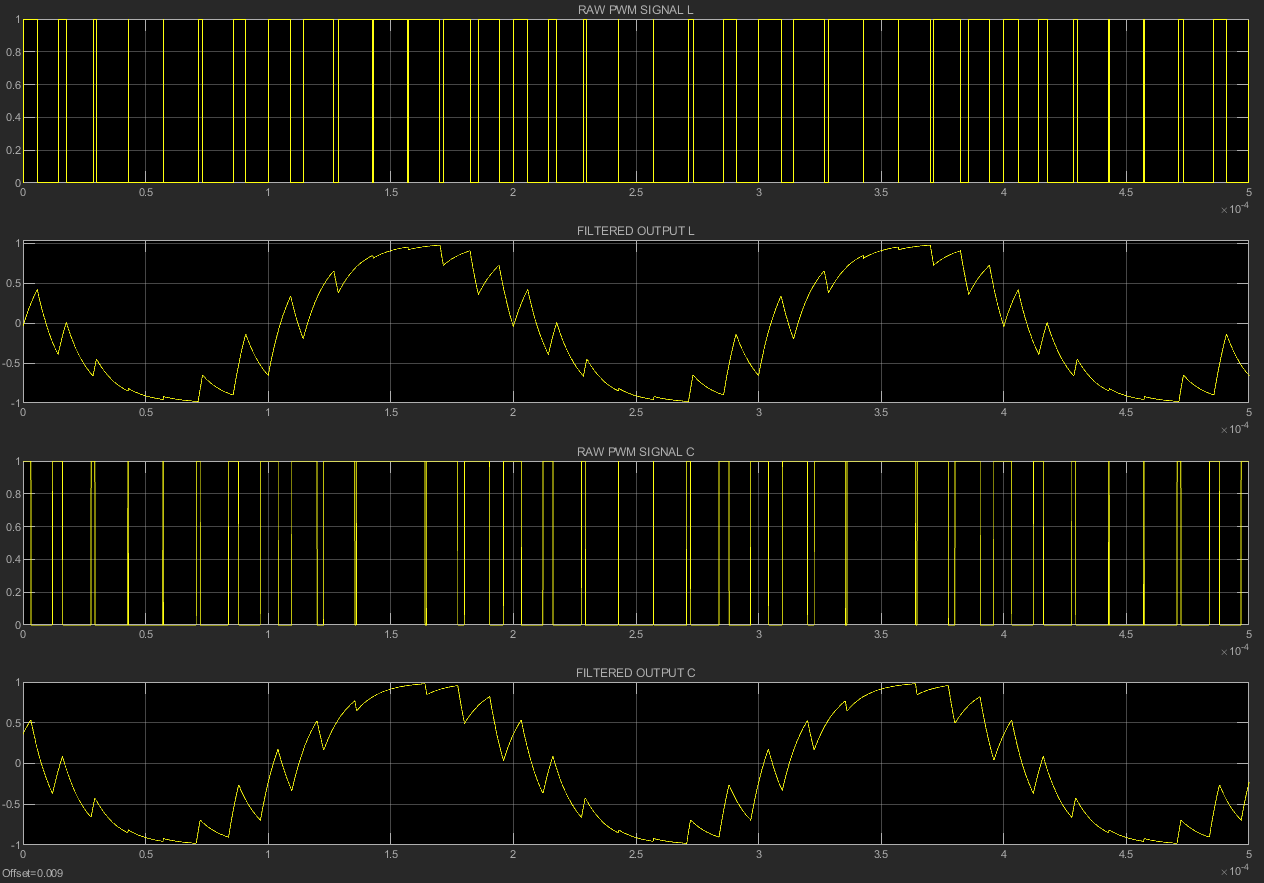As we can see, the output signal is quite similar to a sine wave, but contains “errors” that we don’t want. These are harmonics from the PWM-signal and the imperfect filter. In the time domain, we can’t really see a difference between both PWM modes. Now let’s take a look at both filtered output signals in the frequency domain: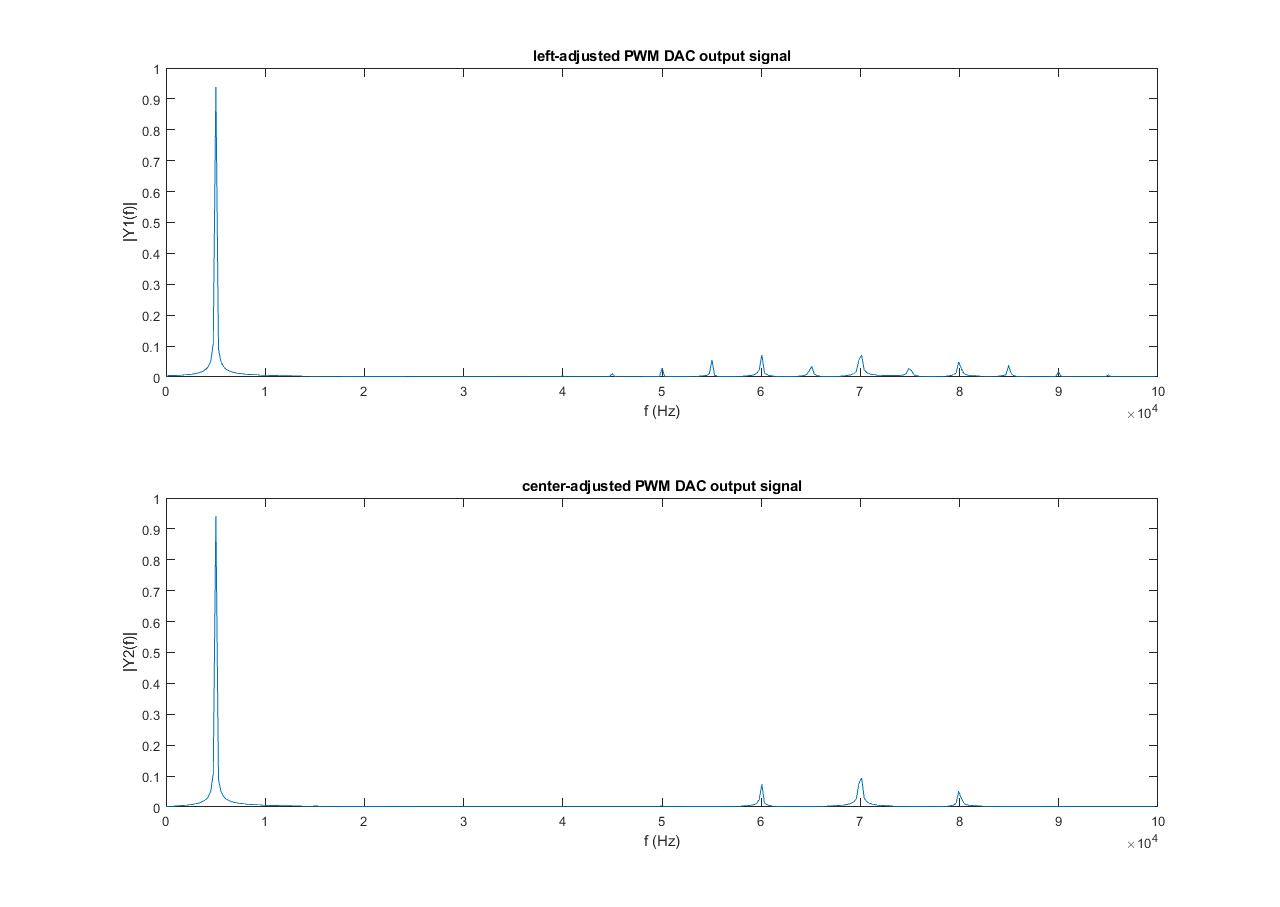This plot shows basically how much each frequency contributes to the signal. We can see a large peak at 5kHz, the frequency of the sine wave we want to create. Now if this sine wave would be ideal, there would only be one peak at exactly 5kHz with an amplitude of 1, and nothing else. But as we can see, there are other, smaller peaks. These are the harmonics which produce the ‘error’ in our signal. The biggest of those peaks is at 70kHz, which is our PWM frequency.

Now in the case of the left-aligned PWM, we have additional harmonics at 70kHz +- multiples of 5kHz, i.e. our PWM frequency and multiples of the input signal frequency. The center-aligned PWM also has harmonics, but fewer: they are located at 70kHz +- multiples of 10kHz, so twice our input signal frequency. We can also see that the lower frequency harmonics, e.g. at 50kHz are considerably smaller than with the left-adjusted PWM.

So we could conclude that center-aligned PWM is always better for such an application… but wait a minute! We already saw that the higher our PWM frequency is w.r.t. our signal frequency, the farther away are the harmonics in the spectrum which leads to a cleaner signal. But in the real application, the maximum PWM frequency we can use is limited by our microcontroller. In case of the left-adjusted PWM, the PWM frequency is basically the timer/counter’s input frequency (e.g. the main clock) divided by the maximum counter value (e.g. 256 for 8bit of resolution). But in case of the centered PWM, the maximum PWM frequency that can be achieved with a given input clock is only half as large. This is because the counter counts upwards and then downwards again instead of counting upwards and then resetting. Therefore, for our analysis to match the real-world situation, we must compare the centered PWM mode, with the left-adjusted mode with twice the frequency. Here we can see the results of the simulation of the centered PWM with 70kHz and a left-adjusted PWM with 140kHz. The filter is the same as before.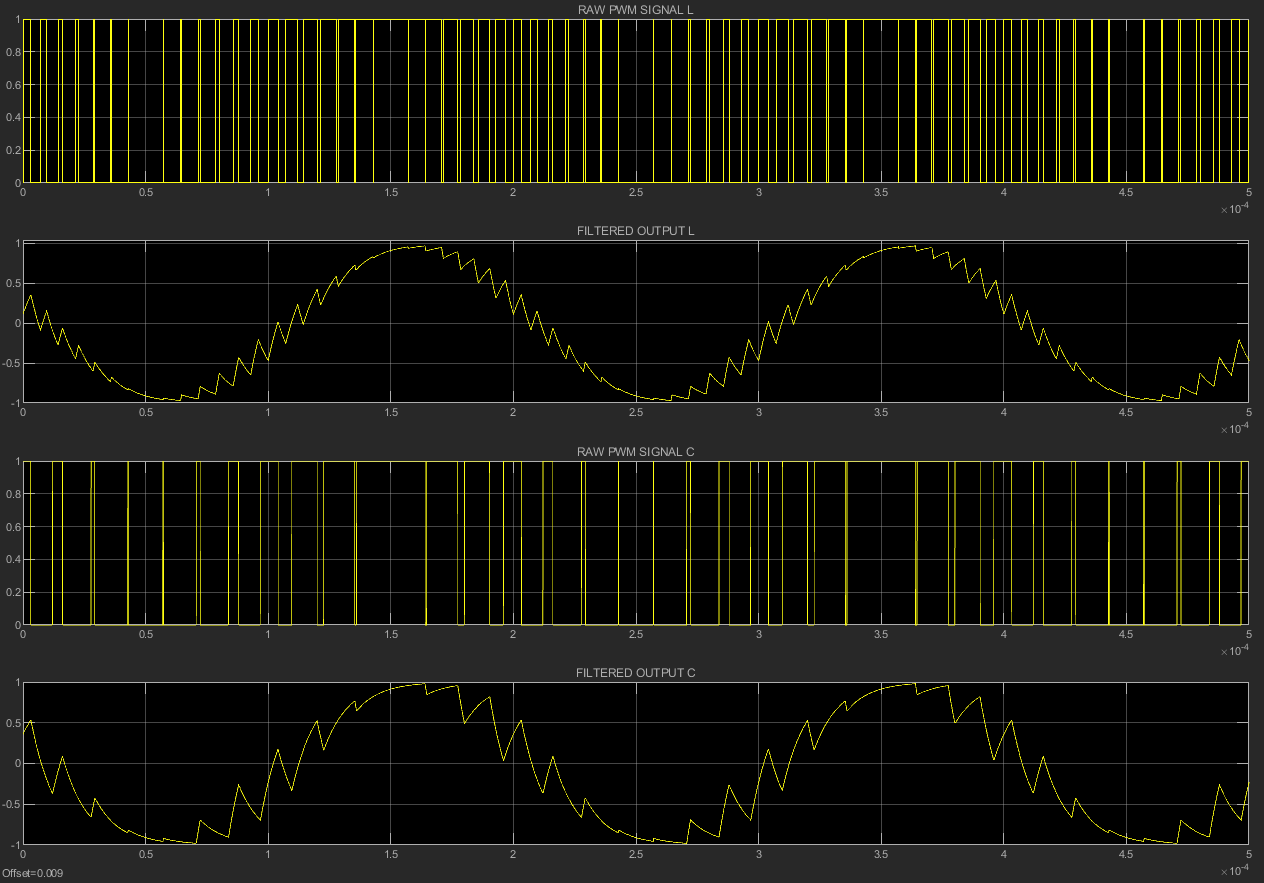We can clearly see the signal created by the left-adjusted PWM being much smoother. Taking a look at the frequency spectrum confirms this: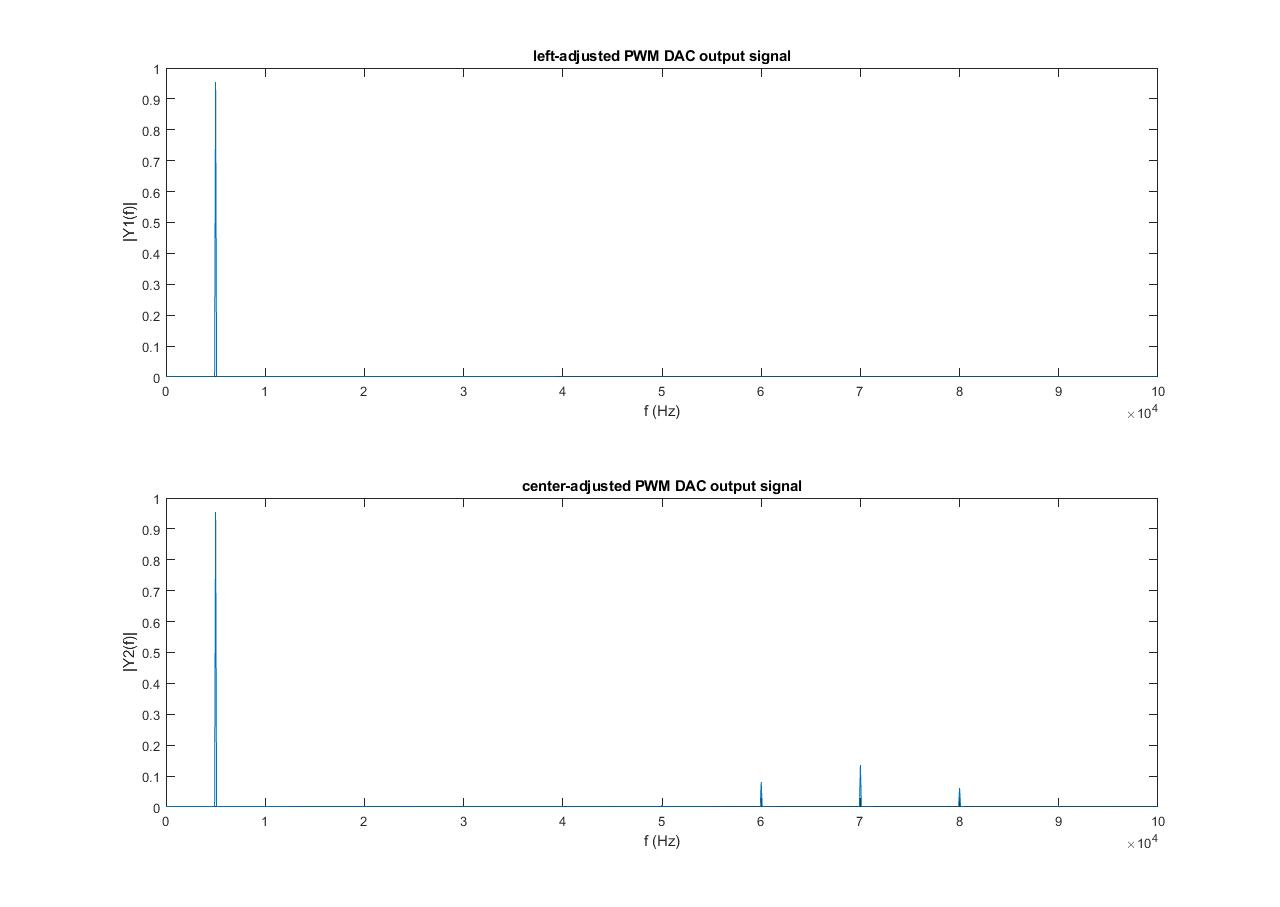Since the left-adjusted PWM’s frequency is now twice higher (140kHz), the harmonics are shifted to the left in the spectrum. So if our application is e.g. to create an audio signal, this is good because there are less harmonics in the audible range. But there are other applications, e.g. in power electronics where this is not advantageous because the harmonics are only shifted to the right in the spectrum, but not lessened.

Therefore we can conclude two things:

• there is a trade-off between the counter resolution and the achievable PWM frequency: if we use a low maximum value for the counter (e.g. 8bit vs 16bit), we have a low resolution to quantisize the amplitude (i.e. we have less ‘steps’ for the duty cycle), but we can achieve a higher PWM-frequency which results in less harmonics and vice-versa
• whether centered or left-aligned PWM mode is better depends on the application. Centered mode produces less harmonics, but has a smaller maximum frequency, thus the harmonics are closer to our main signal in the spectrum.

E.g. to create an audio signal, left-aligned PWM is better because we can use a higher resolution or PWM frequency, this advantage outweighs the higher harmonics. In power electronics applications, centered PWM mode is better. When driving MOSFETs, the losses scale with the switching frequency. Since centered mode has less harmonics right of the PWM frequency (in the spectrum) there is less HF-content and thus there are less power losses in the driver.

That’s all for now, in the next post I’ll show how I implemented this method on a STM32F103 microcontroller to play an audio sound using PWM. Stay tuned!

Written on February 28, 2019